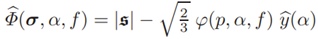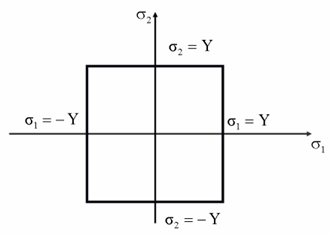top of page
Search

# Brittle And Ductile Failure Theories In FEA - Which Ones Should We Choose?

All engineering materials like metals, plastics, glass, and concrete have limited lifetime. Various factors like fatigue, corrosion, erosion, stress corrosion cracking or foreign object damage effect the strength, stability, and chemical composition of the material leading to its failure. This post is aimed at summarizing some of the more common failure theories and providing guidance on which to use depending on material type...

## Introduction

Out of all external factors corrosion and mechanical failure are the leading causes for material failure. Mechanical failure is typically classified into two types as follows:

1. Ductile Failure – This failure occurs in metals which undergo substantial deformation before fracture. There is huge plastic deformation and visible necking in the material before fracture.

2. Brittle Failure – This type of failure occurs in materials like plastic, rock, concrete, glass and composites. The materials undergo sudden fracture without any noticeable deformation. There is very small or no plastic deformation before fracture in these materials.

Predicting failure of a specimen subjected to uniaxial loading is straight forward. It fails when the uniaxial stress reaches yield strength in ductile materials and ultimate strength in brittle materials. But when the specimen is subjected to complex loading, predicting failure is difficult. There is no universal failure theory which is applicable to all materials and the predictions from failure theories must be consistent with the experimental observations. This article discusses different failure theories for ductile and brittle material. The yield surfaces for plane stress conditions are compared between different failure theories.

## Failure Theories For Ductile Materials

The yielding will not be caused by hydrostatic stresses in the ductile material. The deviatoric stresses causes shape distortions by shearing the material. This deviatoric stresses are responsible for yielding in ductile materials. A good failure theory for ductile materials must take only deviatoric stress into to account for determining fracture.

### Tresca Criterion (Maximum Shear Stress)

This theory states that failure occurs in a material when the absolute value of maximum shear stress reaches the shear stress at yielding in a simple uniaxial tension test.This theory is consistent with failure being independent of hydrostatic stress. In case of plane stress, the yield surface for Tresca criteria is represented in principal stress space as shown in figure below.### Von Mises Yield Criterion (Maximum Distortion Energy)

This theory states that failure occurs in the material when maximum distortion energy is equal to distortion energy at yielding in a uniaxial tension test. The distortion energy is caused by shear stresses present in the material. This is calculated using principal stresses as:In a uniaxial tension test, the distortion energy at yielding is calculated as,From the above equations, the failure criteria is represented mathematically as,This yield surface is elliptical in plane stress condition. Von Mises theory agrees better with the experimental data. Tresca yield surface is encompassed by von Mises yield surface. Hence, Tresca is more conservative failure criterion.To learn more about the Von Mises yield criterion, check out this post that we previously posted on the topic!

### Hosford Yield Criterion

This is a failure criterion used to determine the onset of plastic deformation in a material under triaxial stress state. It is mathematically expressed as,when a=1, the above represents Tresca criterion and a=2 represents Von Mises yield criterion. This is considered as an interpolation between Tresca and Von Mises yield surfaces as shown in the figure below.### Gurson Failure Criterion

This yield criterion is applicable for ductile plastic porous solids with general ellipsoidal voids. Mathematically, this failure theory is represented as,Von Mises theory differs from Gurson by the pressure dependent function ϕ(p, α, f) which is expanded as,When f=0 in the above equation, it reverts to von Mises criterion. The material has yield stress in terms of one-dimensional initial yield stress y0, associated strain α0, Youngs modulus E, hardening exponent H and equivalent plastic strain α as,The yield surface for different values of void volume fraction f versus von Mises yield surface with f=0 is shown below.## Failure Theories For Brittle Materials

Brittle materials fail due to fracture rather than yielding. In these materials, the compressive strengths are higher than tensile strengths. So, the failure theories must capture this difference in tension and compression.

### Rankine Failure Theory (Maximum Principal Stress)

This theory assumes that failure in the material occurs when maximum or minimum principal stress reaches failure strength of the material (σu).This is a simple theory, but it does not consider that yielding is only dependent on deviatoric stress. Hence, this is only suitable for brittle materials and not for ductile materials.### Coulomb-Mohr Failure Criterion

This theory considers the sensitivity of failure to hydrostatic stress and accounts for the difference between compressive and tensile fracture strengths. This assumes that the plane of maximum and minimum principal stresses contains the normal to the plane of failure. Mathematically, this theory is expressed as,In plane stress condition, the yield surface is represented as shown in the figure below.### Modified Mohr Failure Criterion

The Coulomb-Mohr theory does not agree well with experimental data. So, a modification is proposed requiring 3 material constants. This is preferred theory for different types of soils. It captures pressure dependent shear strength, irrecoverable compaction and nonlinear elastic unloading in brittle materials. Mathematically, this is same as Mohr equation with modification represented as,In plane stress condition, the yield surface is represented as shown in the figure below.### Final Thoughts

It is very important to understand the magnitude of stresses required in an object for it to fail. Hopefully this article has provided good insight into the difference between ductile and brittle failure theories. They help in predicting life and functionality of different mechanical and physical components subjected to loading.

If you need help with FEA or simulation in general, don’t hesitate to give us a call! We’re passionate about what we do, and we would love to learn more about your unique engineering challenges.

8,157 views
bottom of page